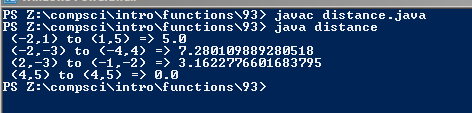# Assignment #93 and Distance Formula

## Code

```        //Name: Mark Katz
///Period: 6
///Program Name: Distance Formula
///File Name: distance.java
///Date Finished: 11/17/15
public class distance
{
public static void main( String[] args )
{

double d1 = distance(-2, 1, 1, 5);
System.out.println(" (-2,1) to (1,5) => " + d1 );

double d2 = distance(-2,-3 , -4,4);
System.out.println(" (-2,-3) to (-4,4) => " + d2 );

System.out.println(" (2,-3) to (-1,-2) => " + distance(2,-3,-1,-2) );

System.out.println(" (4,5) to (4,5) => " + distance(4,5,4,5) );
}

public static double distance(  int x1, int y1, int x2, int y2 )
{
double D = Math.sqrt(((x2 - x1)*(x2 - x1)) + ((y2 - y1)*(y2 - y1)));
return D;

}
}
```

### Picture of the output